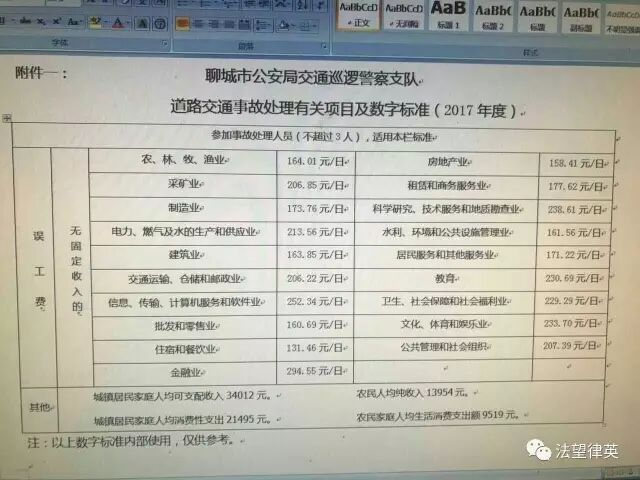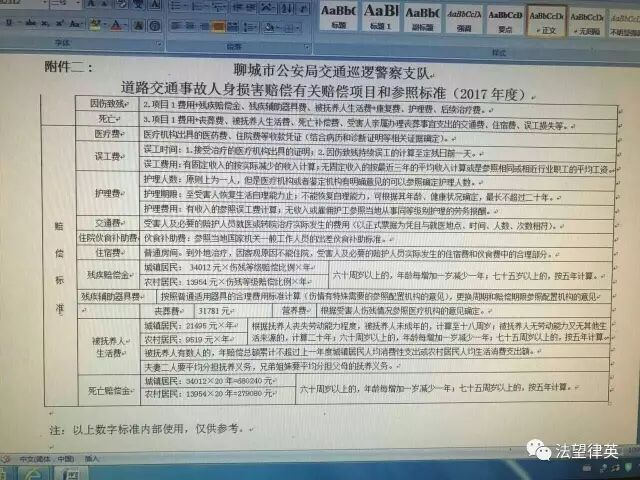您的当前位置： 首页 >> 交通事故 >> 交通赔偿常识 >> 文章正文
 2017年度山东省人身损害赔偿常用数字 阅读选项: 自动滚屏[左键停止] 作者:  来源:  阅读: 一、丧葬费：31781元二、死亡赔偿金：农村;13954×20=279080元城镇；34012×20=680240元60岁以上60岁  农村13954×20=279080元      城镇34012×20=680240元61岁  农村13954×19=265126元      城镇34012×19=646228元62岁  农村13954×18=251172元      城镇34012×18=612216元63岁  农村13954×17=237218元      城镇34012×17=578204元64岁  农村13954×16=223264元     城镇34012×16=544192元65岁  农村13954×15=209310元      城镇34012×15=510180元66岁  农村13954×14=195356元      城镇34012×14=476168元67岁  农村13954×13=181402元      城镇34012×13=442156元68岁  农村13954×12=167448元      城镇34012×12=408144元69岁  农村13954×11=153494元      城镇34012×11=374132元70岁  农村13954×10=139540元      城镇34012×10=340120元71岁  农村13954×9=125586元       城镇34012×9=306108元72岁  农村13954×8=111632元       城镇34012×8=272096元73岁  农村13954×7=97678元       城镇34012×7=238084元74岁  农村13954×6=83724元       城镇34012×6=204072元75岁及以上 农村13954×5=69770元       城镇34012×5=170060元 三、被抚养人生活费：未成年人;0岁  农村9519×18年=171342元      城镇 21495×18=386910元1岁  农村9519×17年=161823元      城镇 21495×17=365415元2岁  农村9519×16年=152304元      城镇 21495×16=343920元3岁  农村9519×15年=142785元      城镇 21495×15=322425元4岁  农村9519×14年=133266元       城镇 21495×14=300930元5岁  农村9519×13年=123747元      城镇 21495×13=279435元6岁  农村9519×12年=114228元      城镇 21495×12=257940元7岁  农村9519×11年=104709元      城镇 21495×11=236445元8岁  农村9519×10年=95190元       城镇 21495×10=214950元9岁  农村9519×9年=85671元        城镇 21495×9=193455元10岁 农村9519×8年=76152元       城镇 21495×8=171960元11岁 农村9519×7年=66633元      城镇 21495×7=150465元12岁 农村9519×6年=57114元      城镇 21495×6=128970元13岁 农村9519×5年=47595元      城镇 21495×5=107475元14岁 农村9519×4年=38076元      城镇 21495×4=85980元15岁 农村9519×3年=28557元      城镇 21495×3=64485元16岁 农村9519×2年=19038元      城镇 21495×2=42990元17岁 农村9519×1年=9519元       城镇 21495×1=21495元超过60周岁以上;60岁 农村9519×20年=190380元    城镇 21495×20=429900元61岁 农村9519×19年=180861元    城镇 21495×19=408405元62岁 农村9519×18年=171342元    城镇 21495×18=386910元63岁 农村9519×17年=161823元    城镇 21495×17=365415元64岁 农村9519×16年=152304元    城镇 21495×16=343920元65岁 农村9519×15年=142785元    城镇 21495×15=322425元66岁 农村9519×14年=133266元    城镇 21495×14=300930元67岁 农村9519×13年=123747元    城镇 21495×13=279435元68岁 农村9519×12年=114228元    城镇 21495×12=257940元69岁 农村9519×11年=104709元    城镇 21495×11=236445元70岁 农村9519×10年=95190元     城镇 21495×10=214950元71岁 农村9519×9年=85671元      城镇 21495×9=193455元72岁 农村9519×8年=76152元      城镇 21495×8=171960元73岁 农村9519×7年=66633元      城镇 21495×7=150465元74岁 农村9519×6年=57114元      城镇 21495×6=128970元75岁及以上 农村9519×5年=47595元      城镇 21495×5=107475元四、伤残赔偿金一级伤残农村13954×20×100%=279080      城镇34012×20×100%=680240元二级伤残农村13954×20年×90%=251172      城镇34012×20×90%=612216元三级伤残农村13954×20年×80%=223264      城镇34012×20×80%=544192元四级伤残农村13954×20年×70%=195356      城镇34012×20×70%=476168元五级伤残农村13954×20年×60%=167448      城镇34012×20×60%=408144元六级伤残农村13954×20年×50%=139540      城镇34012×20×50%=340120元七级伤残农村13954×20年×40%=111632      城镇34012×20×40%=272096元八级伤残农村13954×20×30%=83724       城镇34012×20×30%=204072元九级伤残农村13954×20×20%=55816        城镇34012×20×20%=136048元十级伤残农村13954×20×10%=27908       城镇34012×20×10%=68024元60岁以上的，年龄每增加一岁减少一年，七十五以上的，按五年计算。五、农村或农业人员误工费每日计算标准为（本标准仅适用于聊城地区）【农村居民人均纯收入13954元＋农村居民消费性支出9519元】÷365=64.3元附聊城市交通警察支队赔偿图表两份【大 中 小】【关闭窗口】
 站内搜索
 标 题 内 容
 点击排行

 设为主页  |  收藏本站 | 友情链接 | 管理登录